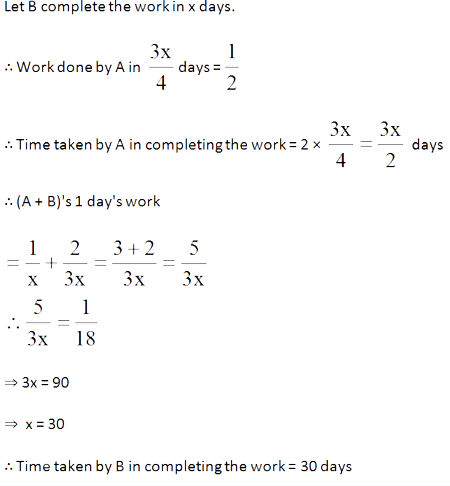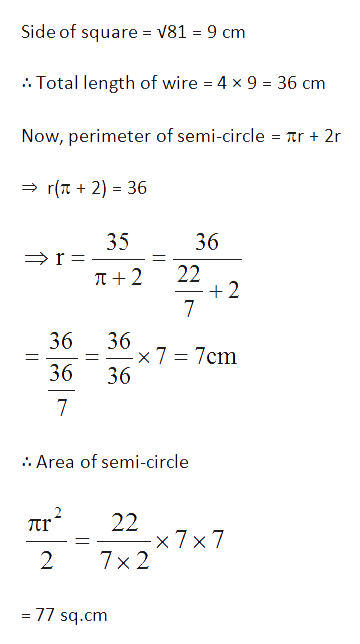# Sample Aptitude Questions of Convergys

1. If A's income is 50% less than that of B's, then B's income is what per cent more than that of A?
1. 125
2. 100
3. 75
4. 50

Required percentage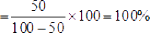1. 1.14 expressed as a per cent of 1.9 is
1. 6%
2. 10%
3. 60%
4. 90%

Required percentage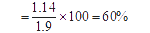2. Two natural numbers are in the ratio 3 : 5 and their product is 2160. The smaller of the numbers is
1. 36
2. 24
3. 18
4. 12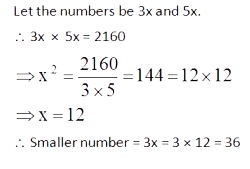3. If 60% of A = ¾ of B, then A : B is
1. 9 : 20
2. 20 : 9
3. 4 : 5
4. 5 : 4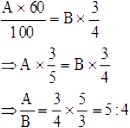4. Two successive price increases of 10% and 10% of an article are equivalent to a single price increase of
1. 19%
2. 20%
3. 21%
4. 22%

Single equivalent percentage increase in price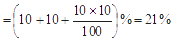5. If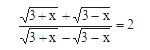then x is equal to
1. 5/12
2. 12/5
3. 5/7
4. 7/5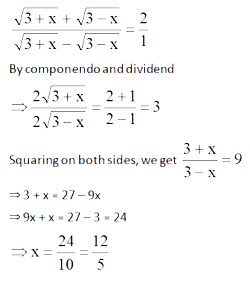6. An equilateral triangle of side 6 cm has its corners cut off to form a regular hexagon. Area (in cm2) of this regular hexagon will be
1.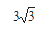2.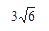3.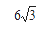4.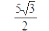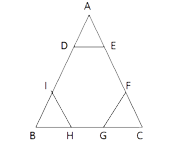side of regular hexagon =1/3 *6 = 2 cm
Area of the hexagon = 3 √3/ 2 a2
= 3 √3/2 *2*2 =6 √3 sq.cm
7. A and B together can do a work in 10 days. B and C together can do the same work in 6 days. A and C together can do the work in 12 days. Then A, B and C to¬gether can do the work in
1. 28 days
2. 14 days
3. 5 5/7 days
4. 8 2/7 days

(A+B)' 1 day's work = 1/10
(B+C) 1 day's work = 1/6
(C+ A) 1 day's work = 1/12
2(A+B+C)' 1 day's work
=1/10 + 1/6 + 1/12 = 6+10+5 / 60
=21/60 = 7/20
=>(A+B+C)' 1 day's work = 7/40
=>All three together will complete the work in 40/7  = 5 5/7days.
8. A does half as much work as B in three- fourth of the time. If together they take 18 days to complete a work, how much time shall B take to do it alone?
1. 30 days
2. 35 days
3. 40 days
4. 45 days